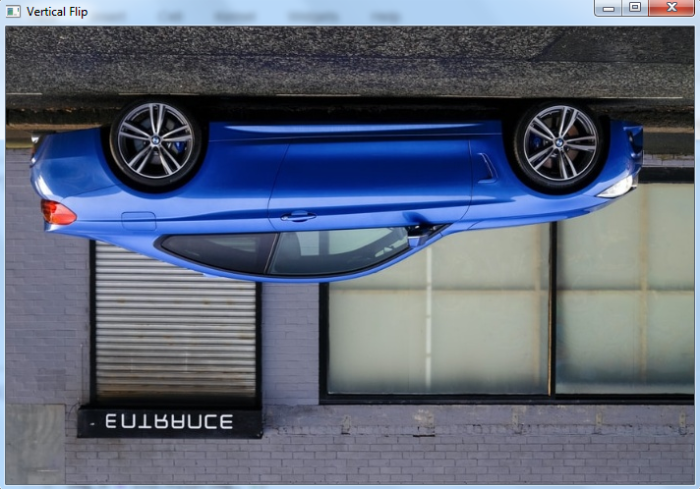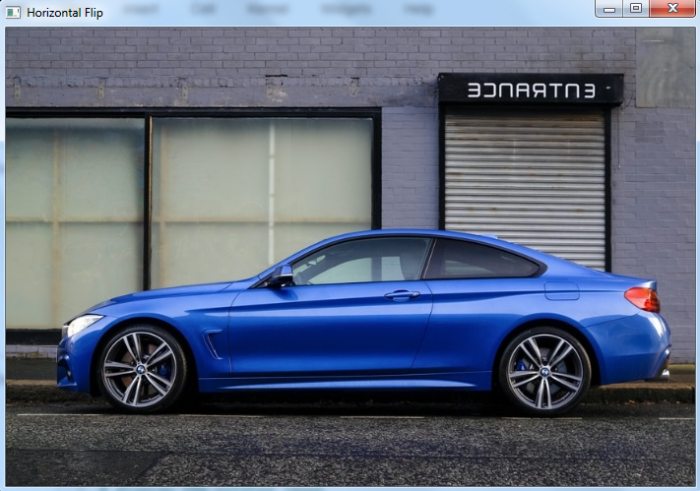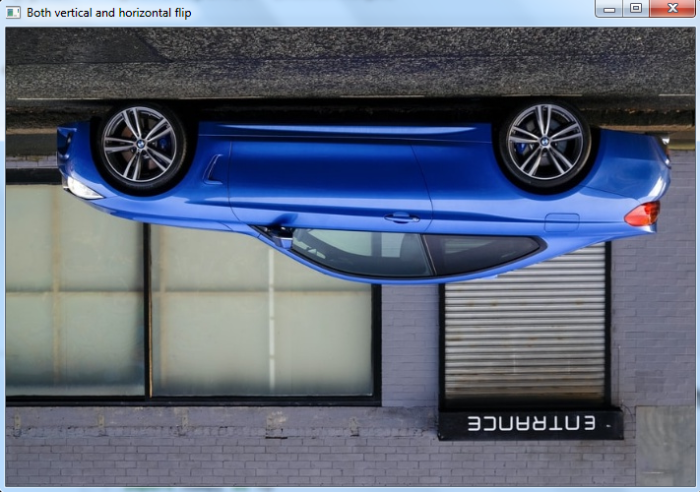# How to flip an image in OpenCV Python?

In OpenCV, an image can be flipped using the function cv2.flip(). Using this function we can flip the image across X-axis, Y-axis and across both axes. It accepts a flag flipCode as an argument to flip the image across the axis.

If the flipCode is set to 0, the image is flipped across the x-axis and if the flipCode is set to a positive integer (say 1), the image is flipped across the Y-axis. If the flipCode is set to a negative integer (say "-1"), the image is flipped across both axes.

## Steps

To flip an image, one could follow the steps given below −

• Import the required library. In all the following examples, the required Python library is OpenCV. Make sure you have already installed it.

• Read the input image using cv2.imread() method. Specify full path of image with the image type (i.e. png or jpg)

• Apply cv2.flip() function on the input image img. Pass the parameter flipCode for desired flip. We set flipCode=0 to flip around the x-axis.

img_v = cv2.flip(img, 0)

• Display the flipped output image.

We will use this image as the Input File in the following examples −## Example

In this Python program, we flip the input image across x-axis (vertically).

# import required library
import cv2

# flip the image by vertically
img_v = cv2.flip(img, 0)

# display the rotated image
cv2.imshow("Vertical Flip", img_v)
cv2.waitKey(0)
cv2.destroyAllWindows()


## Output

When you run the above program, it will produce the following output window −Notice the output image is flipped across the X-axis.

## Example

In this Python program, we flip the input image across the y-axis (horizontally).

# import required library
import cv2

# flip the image by horizontally
img_h = cv2.flip(img, 1)

# display the rotated image
cv2.imshow("Horizontal Flip", img_h)
cv2.waitKey(0)
cv2.destroyAllWindows()


## Output

When you run the above program, it will produce the following output window −Notice the output image is flipped across the Y-axis.

## Example

In this Python program, we flip the input image across both axes (vertically as well as horizontally).

# import required library
import cv2

# rotate the image both vertically and horizontally
img_vh = cv2.flip(img, -1)

# display the rotated image
cv2.imshow("Both vertical and horizontal flip", img_vh)
cv2.waitKey(0)
cv2.destroyAllWindows()


## Output

When you run the above program, it will produce the following output window −Notice the output image is flipped across the X-axis and well as Y-axis.